2022: SklogWiki celebrates 15 years on-line

# Compressibility

The bulk modulus B gives the change in volume of a solid substance as the pressure on it is changed,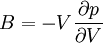$B = -V \frac{\partial p}{\partial V}$

The compressibility K or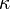$\kappa$, is given by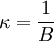$\kappa =\frac{1}{B}$

## Isothermal compressibility

The isothermal compressibility,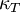$\kappa_T$ is given by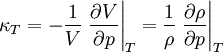$\kappa_T =-\frac{1}{V} \left.\frac{\partial V}{\partial p}\right\vert_{T} = \frac{1}{\rho} \left.\frac{\partial \rho}{\partial p}\right\vert_{T}$

(Note: in Hansen and McDonald the isothermal compressibility is written as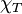$\chi_T$). where$T$ is the temperature,$\rho$ is the particle number density given by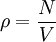$\rho = \frac{N}{V}$

where$N$ is the total number of particles in the system, i.e.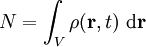$N = \int_V \rho({\mathbf r},t)~{\rm d}{\mathbf r}$

The adiabatic compressibility,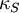$\kappa_S$ is given by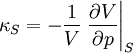$\kappa_S =-\frac{1}{V} \left.\frac{\partial V}{\partial p}\right\vert_{S}$
where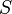$S$ is the entropy.